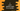# C program to print the addition table for a number### C program to print addition table for a number :

In this C programming tutorial, we will learn how to print the addition table for a specific number.

The user will enter one number, and we will read and save that number. After that, using one loop, the program will print one addition table.

For example, the addition table for 5 will loop like as below :

``````5 + 1 = 6
5 + 2 = 7
5 + 3 = 8
5 + 4 = 9
5 + 5 = 10
5 + 6 = 11
5 + 7 = 12
5 + 8 = 13
5 + 9 = 14
5 + 10 = 15``````

We will learn to solve this problem by using a for loop and by using a while loop. Let’s take a look at the programs:

``````#include <stdio.h>

int main()
{
//1
int number;
int i;

//2
printf("Enter the number : ");
scanf("%d", &number);

//3
for (i = 1; i <= 10; i++)
{
//4
printf("%d + %d = %d\n", number, i, number + i);
}
}``````

### Explanation :

The commented numbers in the above program denote the step number below:

1. Create one variable number to hold the user input number and one variable i to use in the loop below.
2. Ask the user to enter the number. Using scanf, store the user input value to number variable.
3. Run one for loop. This loop will run from i = 1 to i = 10 and increment the value of i by 1 on each iteration of this loop, print one line of the addition table.
4. This printf will print the addition table line. The first %d will print the value of the user-given number, the second %d will print the current value of i, and the third %d will print the value of summation of both values i.e. number + i.

### Sample Output :

``````Enter the number : 6
6 + 1 = 7
6 + 2 = 8
6 + 3 = 9
6 + 4 = 10
6 + 5 = 11
6 + 6 = 12
6 + 7 = 13
6 + 8 = 14
6 + 9 = 15
6 + 10 = 16``````
``````Enter the number : 7
7 + 1 = 8
7 + 2 = 9
7 + 3 = 10
7 + 4 = 11
7 + 5 = 12
7 + 6 = 13
7 + 7 = 14
7 + 8 = 15
7 + 9 = 16
7 + 10 = 17``````

### Using a while loop :

We can achieve the same result by using a while loop also. Take a look at the below program :

``````#include <stdio.h>

int main()
{
int number;
int i;

printf("Enter the number : ");
scanf("%d", &number);

i = 1;
while (i <= 10)
{
printf("%d + %d = %d\n", number, i, number + i);
i++;
}
}``````

Here, instead of a for loop, we are running one while loop to print the addition table. The printf statement is the same as above. Inside the while loop, we are incrementing the value of i each time. It starts from 1 and ends at 10.

The output of this program will look as like below :

``````Enter the number : 9
9 + 1 = 10
9 + 2 = 11
9 + 3 = 12
9 + 4 = 13
9 + 5 = 14
9 + 6 = 15
9 + 7 = 16
9 + 8 = 17
9 + 9 = 18
9 + 10 = 19``````